# The boxplot function in R

## Basic box plot

The `boxplot` function is the function for creating box plots in base R graphics. You can pass a vector or a data frame (to plot all the columns). Since R 4.0.0 the box plots are gray by default, while in previous versions were white.

``````set.seed(7)
x <- rnorm(200)

boxplot(x)``````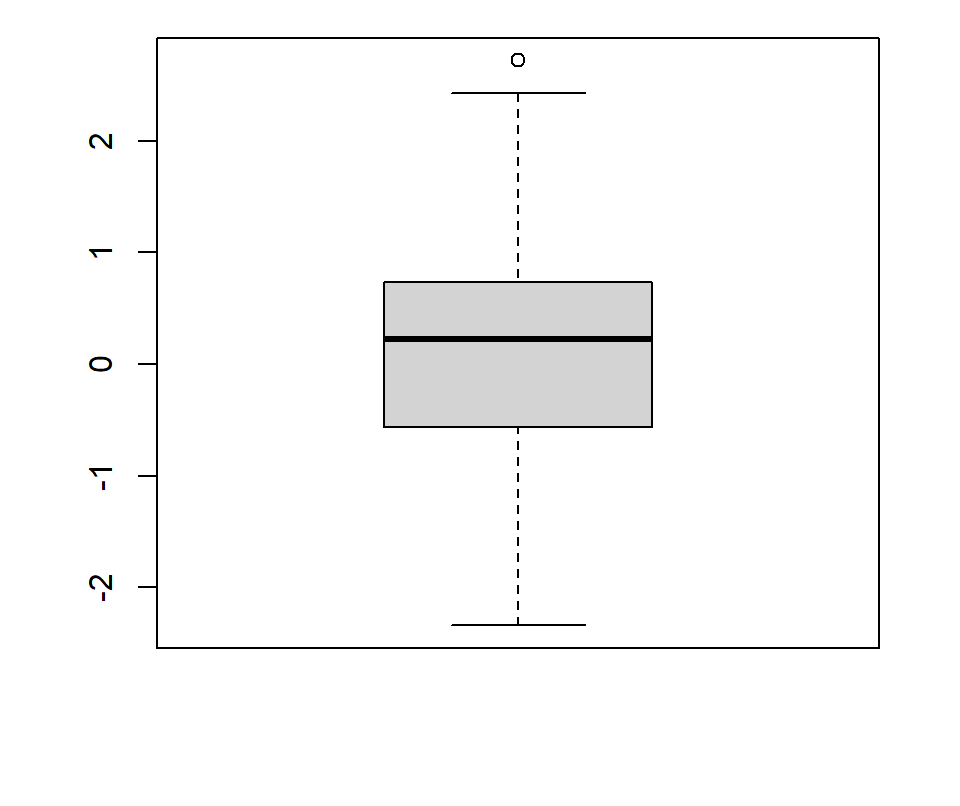Horizontal

The `horizontal` argument can be set to `TRUE` to create a horizontal box plot. The default is vertical.

``````set.seed(7)
x <- rnorm(200)

boxplot(x, horizontal = TRUE)``````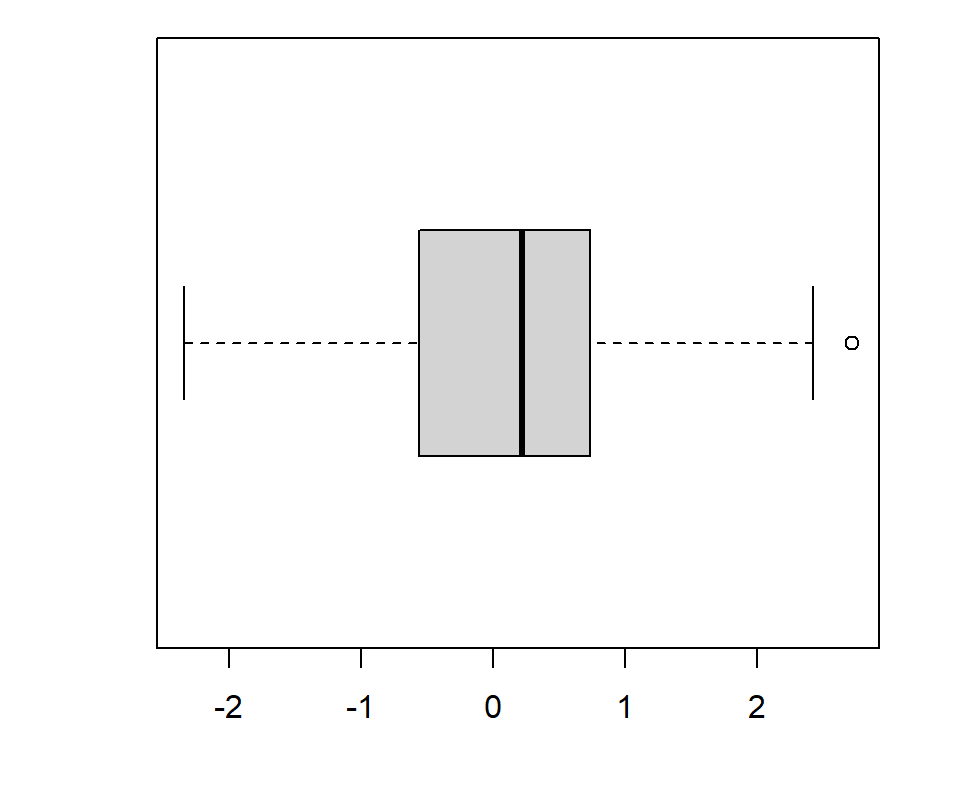Confidence interval (notch)

The function also provides the argument `notch` to add the 95% confidence interval for the median. This is particular interesting for checking if there are evidences that the medians of several box plots differ or not.

``````set.seed(7)
x <- rnorm(200)

boxplot(x, notch = TRUE)``````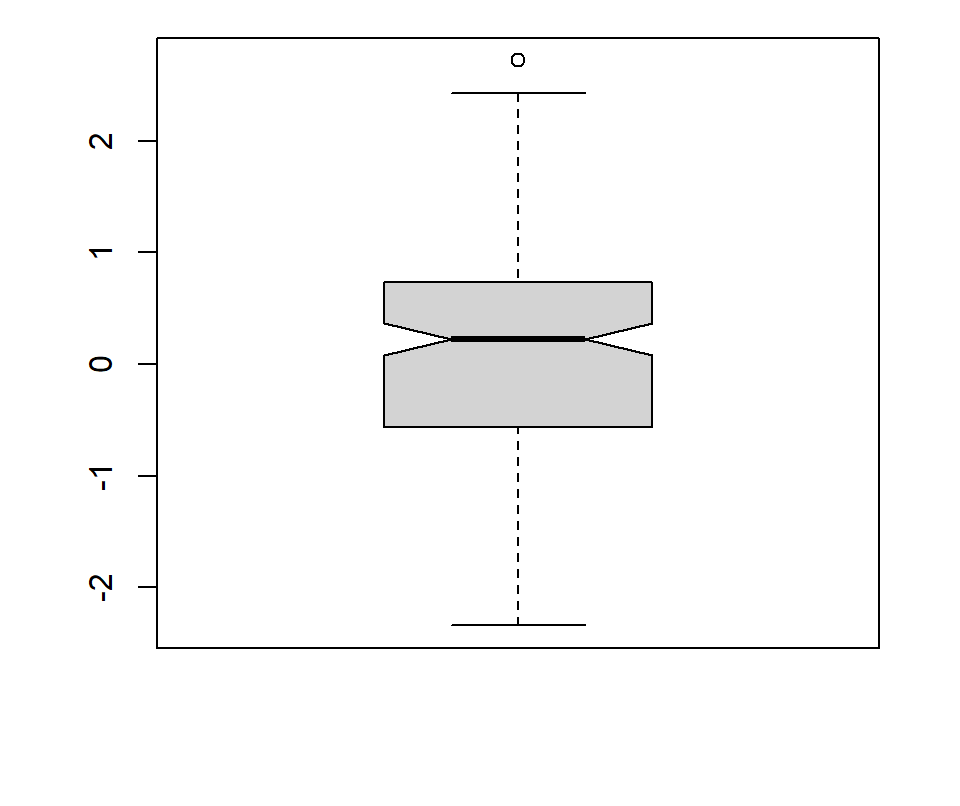Fill color

The `col` argument fills the box with a color. In the following example we are adding a color with transparency making use of the `rgb` function.

``````set.seed(7)
x <- rnorm(200)

boxplot(x, col = rgb(0, 0.5, 1, alpha = 0.5))``````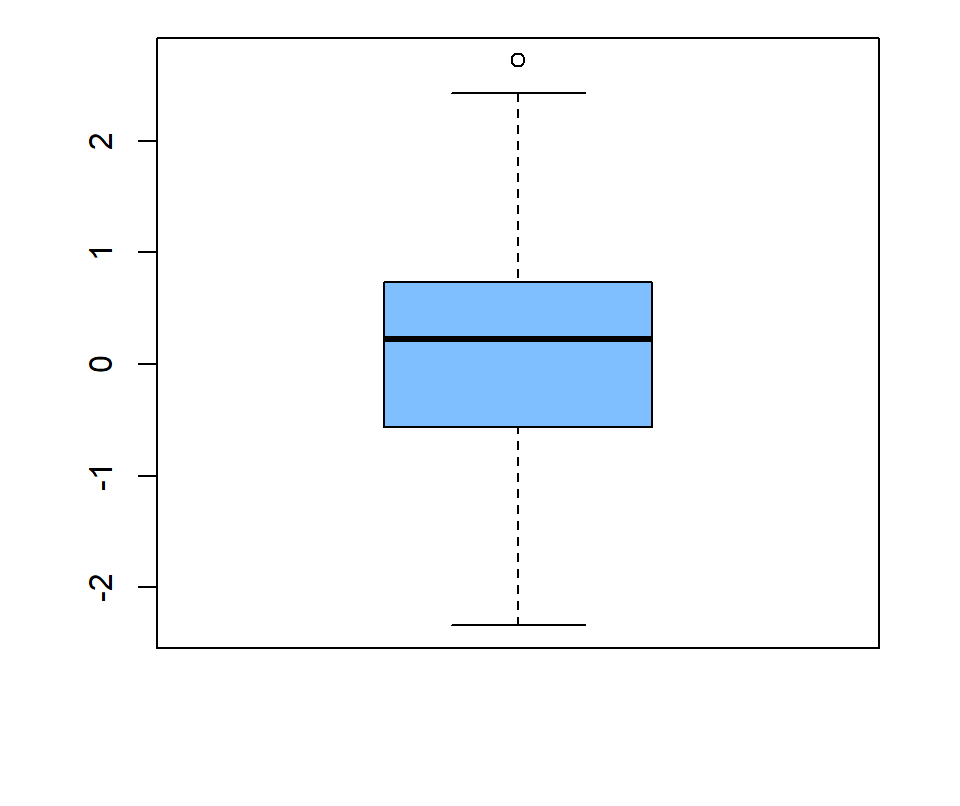Border color

You can also change the color of the border of the whole box plot with `border`.

``````set.seed(7)
x <- rnorm(200)

boxplot(x, col = "white",
border = 4)``````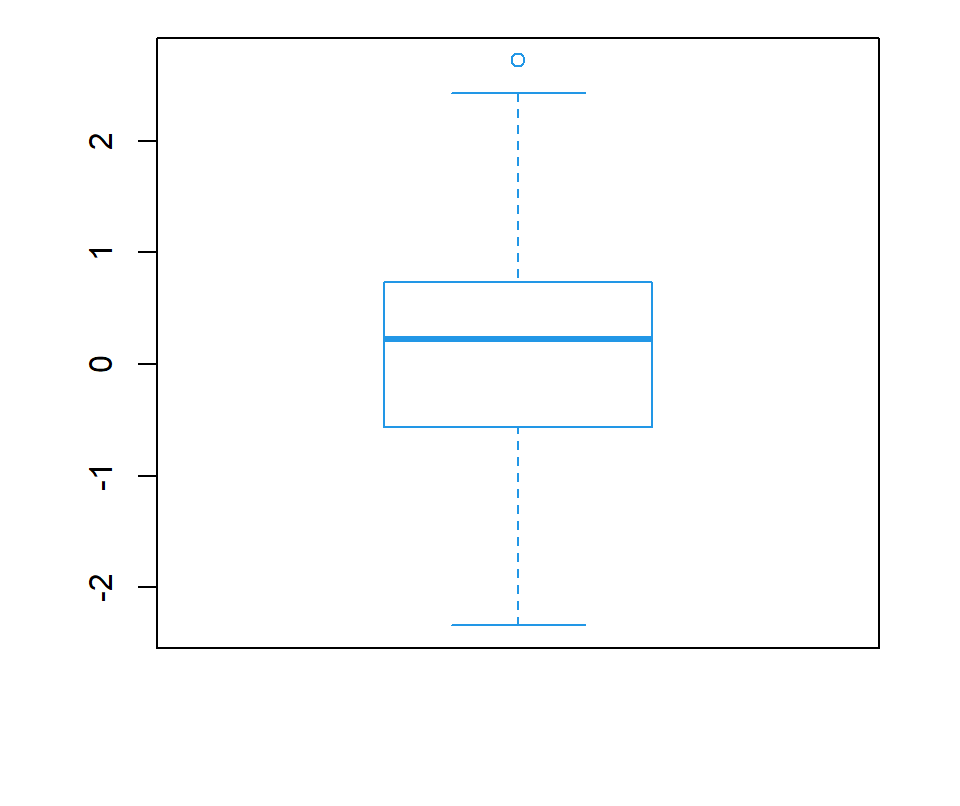Box line type

The `lty` argument controls the line type of all the lines of the box plot.

``````set.seed(7)
x <- rnorm(200)

boxplot(x, col = "white",
lty = 2)``````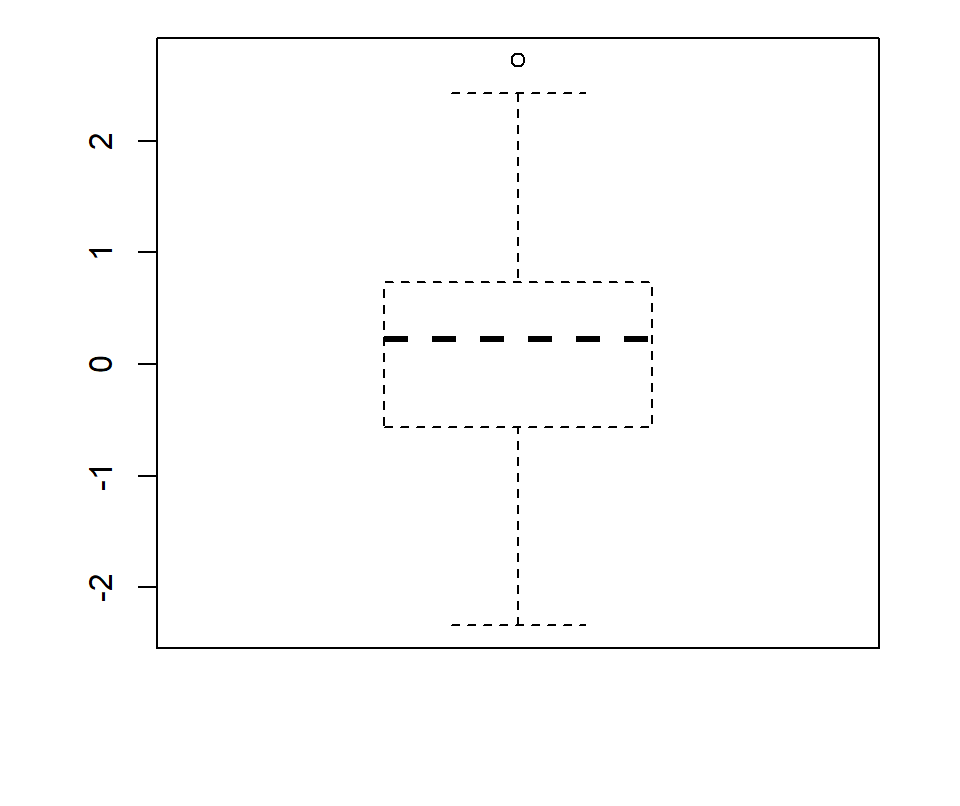## Further customization

The function allows customizing each color and each line individually. Most of the arguments below are part of the `bxp` function, which is called by `boxplot`.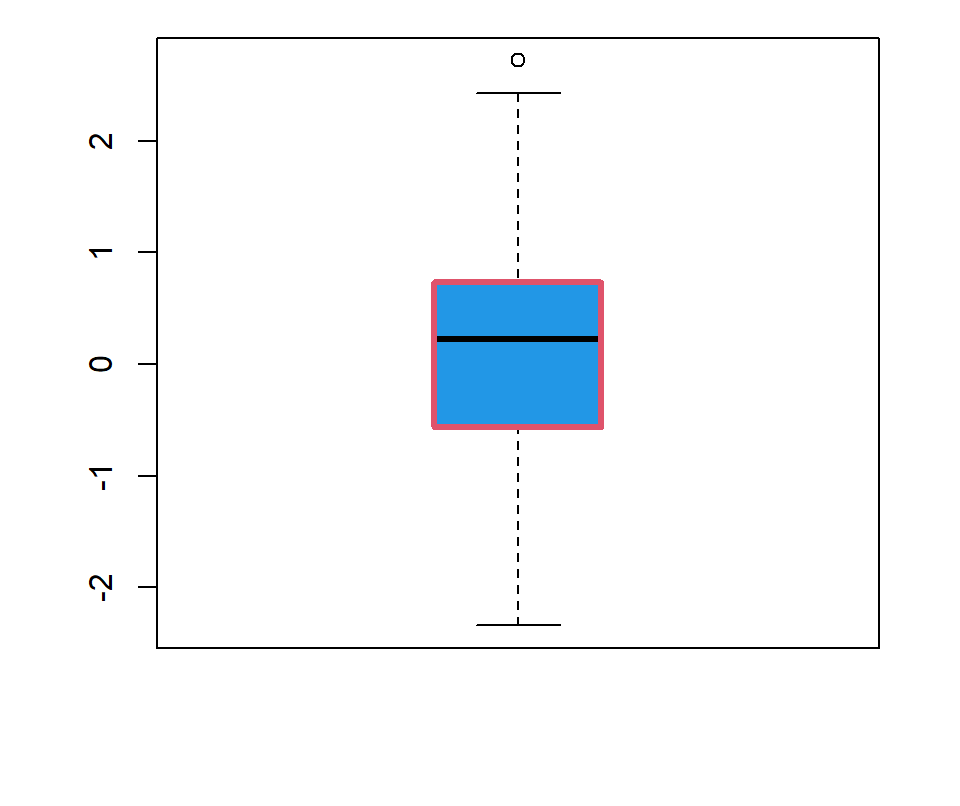Box customization

``````set.seed(7)
x <- rnorm(200)

boxplot(x,
boxwex = 0.5, # Box width
boxlty = 1,   # Box line type
boxlwd = 3,   # Box line width
boxcol = 2,   # Box border color
boxfill = 4)  # Box fill color``````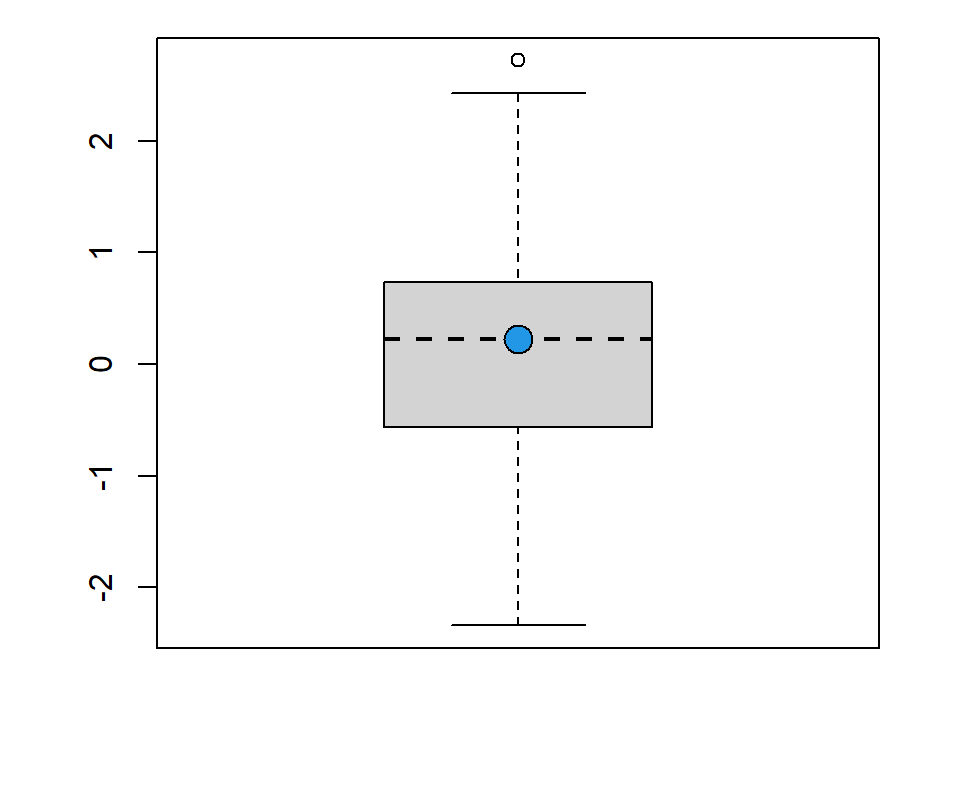Median line customization

``````set.seed(7)
x <- rnorm(200)

boxplot(x,
medlty = 2,  # Line type of the median
medlwd = 2,  # Line width of the median
medpch = 21, # pch symbol of the point
medcex = 2,  # Size of the point
medcol = 1,  # Color of the line
medbg = 4)   # Color of the pch (21 to 25)``````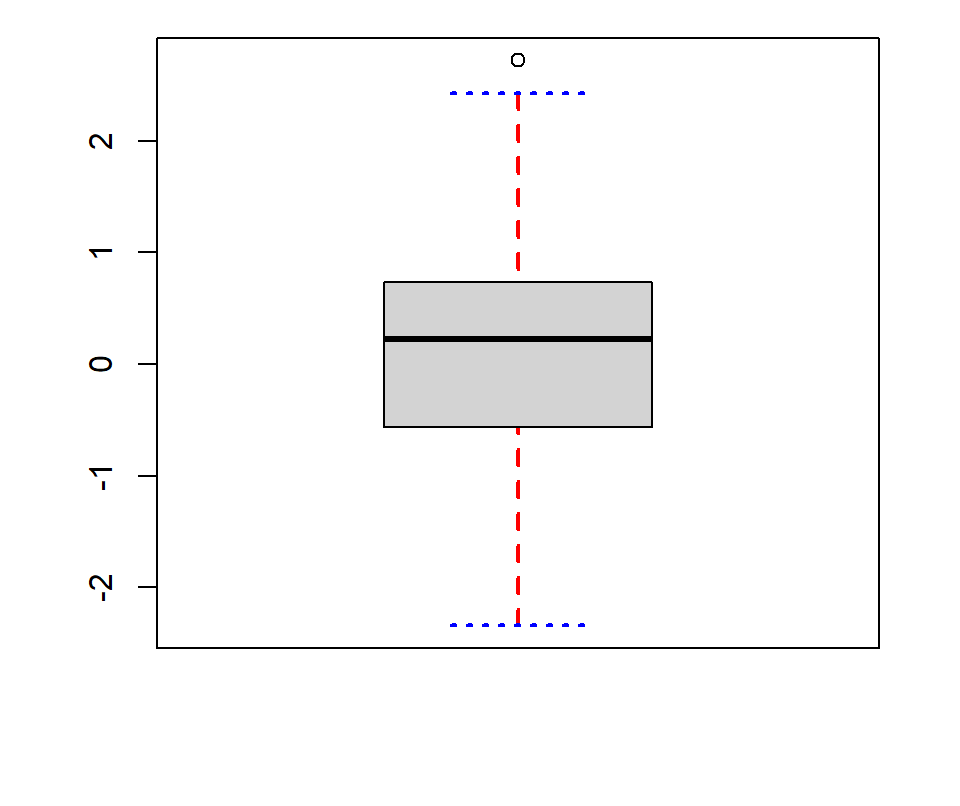Whiskers and staple customization

``````set.seed(7)
x <- rnorm(200)

boxplot(x,
whisklty = 2,       # Line type of the whiskers
whisklwd = 2,       # Line width of the whiskers
whiskcol = "red",   # Color of the whiskers
staplelty = 3,      # Line type of the staples
staplelwd = 2,      # Line width of the staples
staplecol = "blue") # Color of the staples``````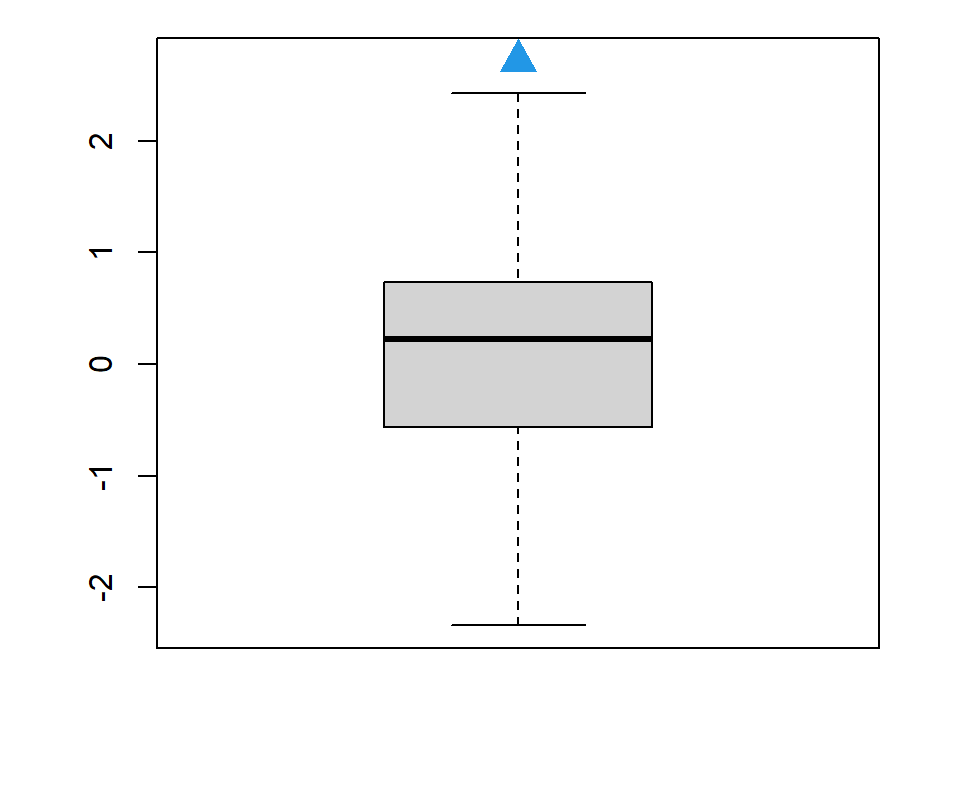Outliers customization

``````set.seed(7)
x <- rnorm(200)

boxplot(x,
outlty = 0,  # Line type
outlwd = 1,  # Line width
outpch = 23, # Pch for the outliers
outcex = 2,  # Size of the outliers
outcol = 1,  # Color
outbg = 4)   # bg color (pch 21 to 25)``````